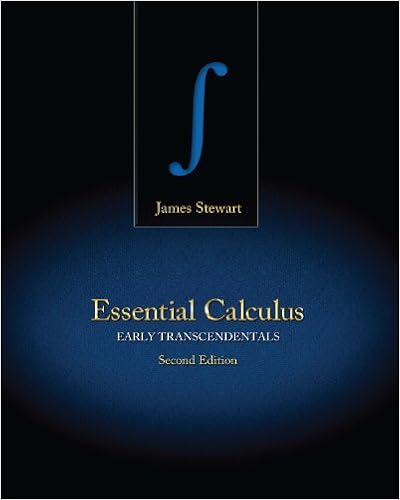# 1st Exam - Exercises - Integration by Parts Trigonometric...

• Test Prep
• 4

This preview shows page 1 - 2 out of 4 pages.

##### We have textbook solutions for you!
The document you are viewing contains questions related to this textbook.The document you are viewing contains questions related to this textbook.
Chapter 6 / Exercise 64
Essential Calculus: Early Transcendentals
StewartExpert Verified
Integration by Parts I. Exercises from the slides: Find the following antiderivatives. 1. Z xe - 2 x dx 2. Z x ln x dx 3. Z (ln x ) 2 dx 4. Z (ln x ) 2 x dx II. Additional exercises 1. Find the following antiderivatives. 1. Z 1 0 sin 2 πx cos 2 πx dx 2. Z cos 3 x sin x dx 3. Z csc 4 x cot 2 x dx 4. Z cos 4 x cos 3 x dx 5. Z tan 3 (ln x ) sec 8 (ln x ) x dx II. Additional exercises 1. Find the following antiderivatives. Trigonometric Integrals I. Exercises from the slides: Evaluate the following integrals.
##### We have textbook solutions for you!
The document you are viewing contains questions related to this textbook.The document you are viewing contains questions related to this textbook.
Chapter 6 / Exercise 64
Essential Calculus: Early Transcendentals
StewartExpert Verified
•••